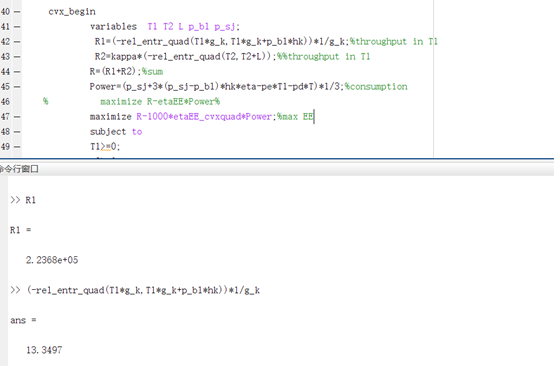# WHY，Who is the best value inCVX，XXXXWhy the two values are not equal after running cvx, R1 is the value generated after cvx compilation, and the following calculation formula is the result of the optimal value operation.
Why is this happening? I’m confused. Can anyone explain this?

Upon CVX cimpletion, presuming it declares optimum, the optimal value is numerically populated in all CVX variables. However, the numerical values of expressions may not necessarily correspond to the optimum,. In your program, `R1` is a CVX expression, and is not a CVX variable.The true optimum is used internally so that the problem is correctly solved, but to be sure you have the correct optimal values, you have to compute the CVX expressions using the CVX (optimal) variable values after CVX execution ends. So 13.3497 is the true optimal value of `R1`, and was used internally in the optimization.

Thank you again,Mark.I understand what caused my wrong idea, the expression is not a variable of cvx, I think this solved one of my problems.